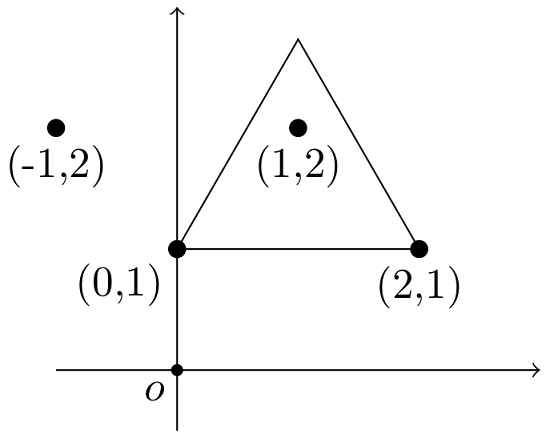Time Limit : sec, Memory Limit : KB

### Equilateral Triangular Fence

Ms. Misumi owns an orchard along a straight road. Recently, wild boars have been witnessed strolling around the orchard aiming at pears, and she plans to construct a fence around many of the pear trees.

The orchard contains n pear trees, whose locations are given by the two-dimensional Euclidean coordinates (x1, y1),..., (xn, yn). For simplicity, we neglect the thickness of pear trees. Ms. Misumi's aesthetic tells that the fence has to form a equilateral triangle with one of its edges parallel to the road. Its opposite apex, of course, should be apart from the road. The coordinate system for the positions of the pear trees is chosen so that the road is expressed as y = 0, and the pear trees are located at y ≥ 1.

Due to budget constraints, Ms. Misumi decided to allow at most k trees to be left outside of the fence. You are to find the shortest possible perimeter of the fence on this condition.

The following figure shows the first dataset of the Sample Input. There are four pear trees at (−1,2), (0,1), (1,2), and (2,1). By excluding (−1,2) from the fence, we obtain the equilateral triangle with perimeter 6.### Input

The input consists of multiple datasets, each in the following format.

n
k
x1 y1
...
xn yn

Each of the datasets consists of n+2 lines. n in the first line is the integer representing the number of pear trees; it satisfies 3 ≤ n ≤ 10 000. k in the second line is the integer representing the number of pear trees that may be left outside of the fence; it satisfies 1 ≤ k ≤ min(n−2, 5 000). The following n lines have two integers each representing the x- and y- coordinates, in this order, of the locations of pear trees; it satisfies −10 000 ≤ xi ≤ 10 000, 1 ≤ yi ≤ 10 000. No two pear trees are at the same location, i.e., (xi, yi)=(xj, yj) only if i=j.

The end of the input is indicated by a line containing a zero. The number of datasets is at most 100.

### Output

For each dataset, output a single number that represents the shortest possible perimeter of the fence. The output must not contain an error greater than 10−6.

### Sample Input

4
1
0 1
1 2
-1 2
2 1
4
1
1 1
2 2
1 3
1 4
4
1
1 1
2 2
3 1
4 1
4
1
1 2
2 1
3 2
4 2
5
2
0 1
0 2
0 3
0 4
0 5
6
3
0 2
2 2
1 1
0 3
2 3
1 4
0


### Output for the Sample Input

6.000000000000
6.928203230276
6.000000000000
7.732050807569
6.928203230276
6.000000000000